# Excel VLOOKUP Function

## VLOOKUP Function

The VLOOKUP function is a premade function in Excel, which allows searches across columns.

It is typed `=VLOOKUP` and has the following parts:

=VLOOKUP(lookup_value, table_array, col_index_num, [range_lookup])

Note: The column which holds the data used to lookup must always be to the left.

Note: The different parts of the function are separated by a symbol, like comma `,` or semicolon `;`

The symbol depends on your Language Settings.

Lookup_value: Select the cell where search values will be entered.

Table_array: The table range, including all cells in the table.

Col_index_num: The data which is being looked up. The input is the number of the column, counted from the left:Range_lookup: TRUE if numbers (1) or FALSE if text (0).

Note: Both 1 / 0 and True / False can be used in Range_lookup.

How to use the VLOOKUP function.1. Select a cell (`H4`)
2. Type `=VLOOKUP`
3. Double click the VLOOKUP command
4. Select the cell where search value will be entered (`H3`)
5. Type (`,`)
6. Mark table range (`A2:E21`)
7. Type (`,`)
8. Type the number of the column, counted from the left (`2`)
9. Type True (1) or False (0) (`1`)
10. Hit enter
11. Enter a value in the cell selected for the Lookup_value `H3(7)`

Let's have a look at an example!

Use the VLOOKUP function to find the Pokemon names based on their ID#: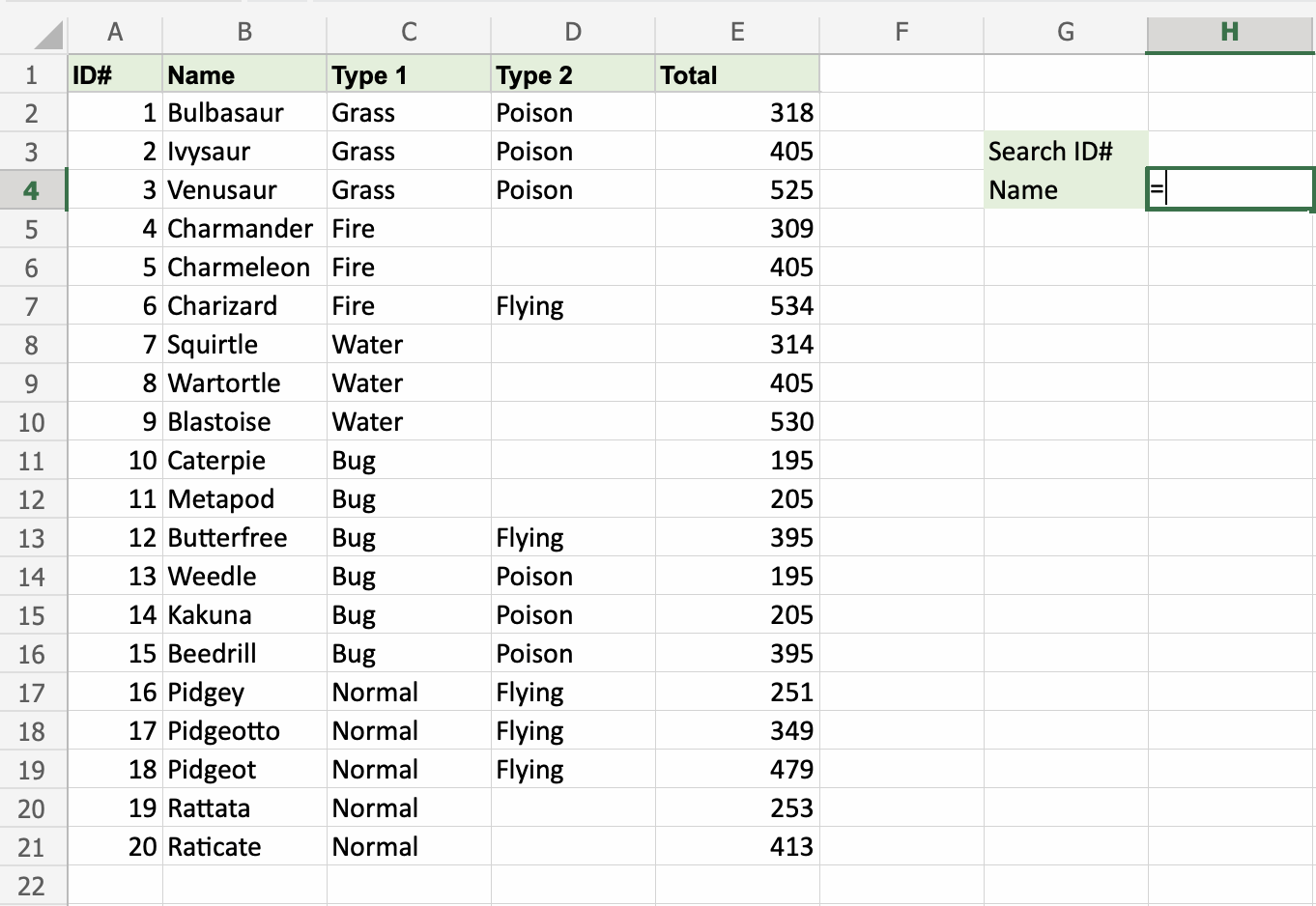`H4` is where the search result is displayed. In this case, the Pokemons names based on their ID#.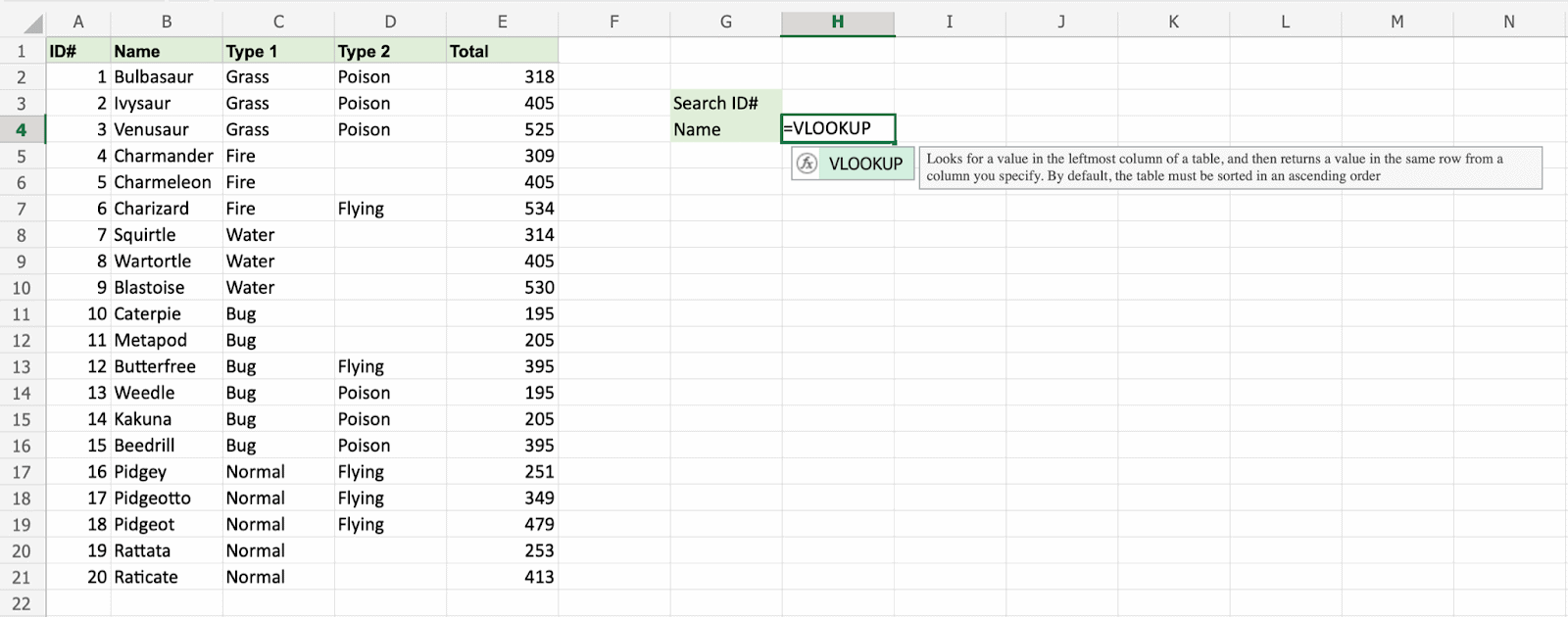`H3` selected as lookup_value. This is the cell where the search query is entered. In this case the Pokemons ID#.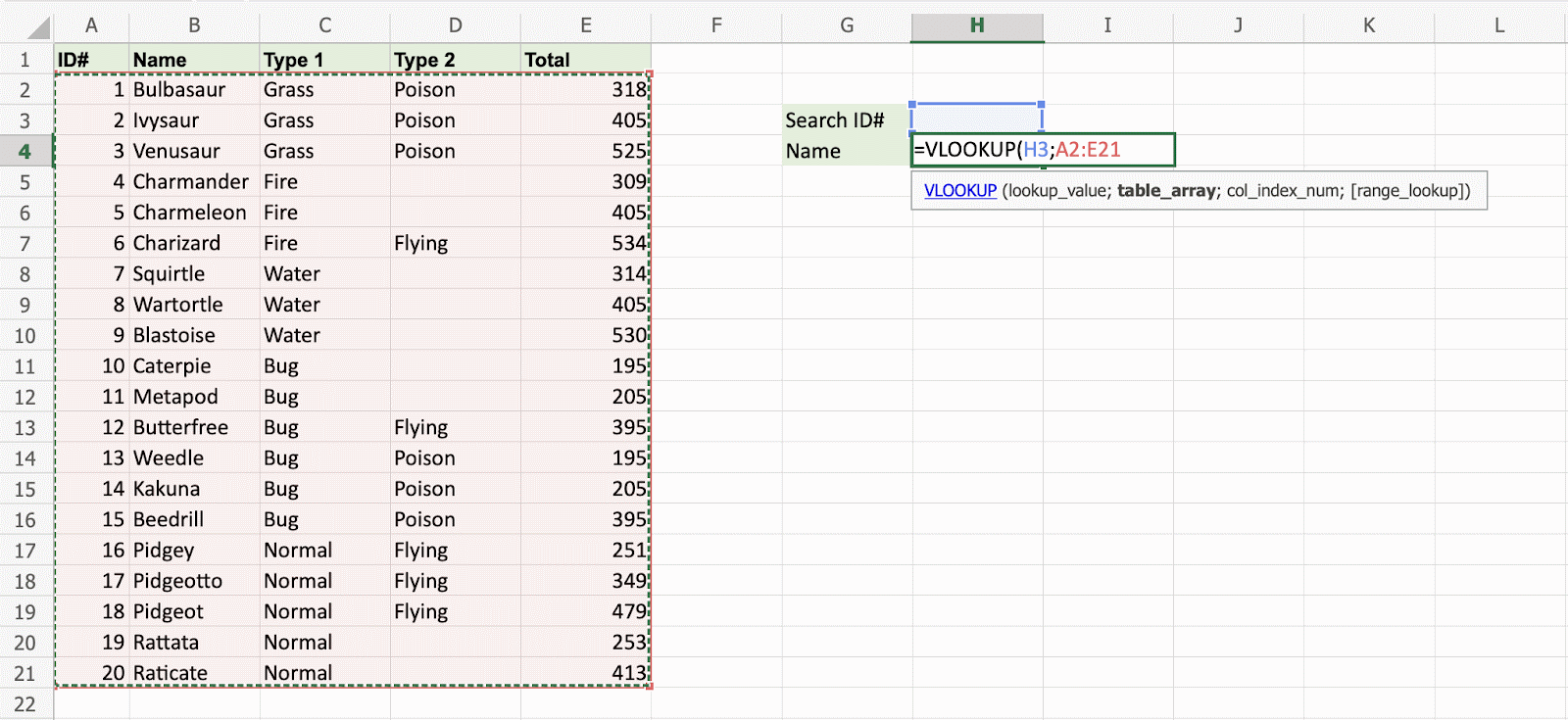The range of the table is marked at table_array, in this example `A2:E21`.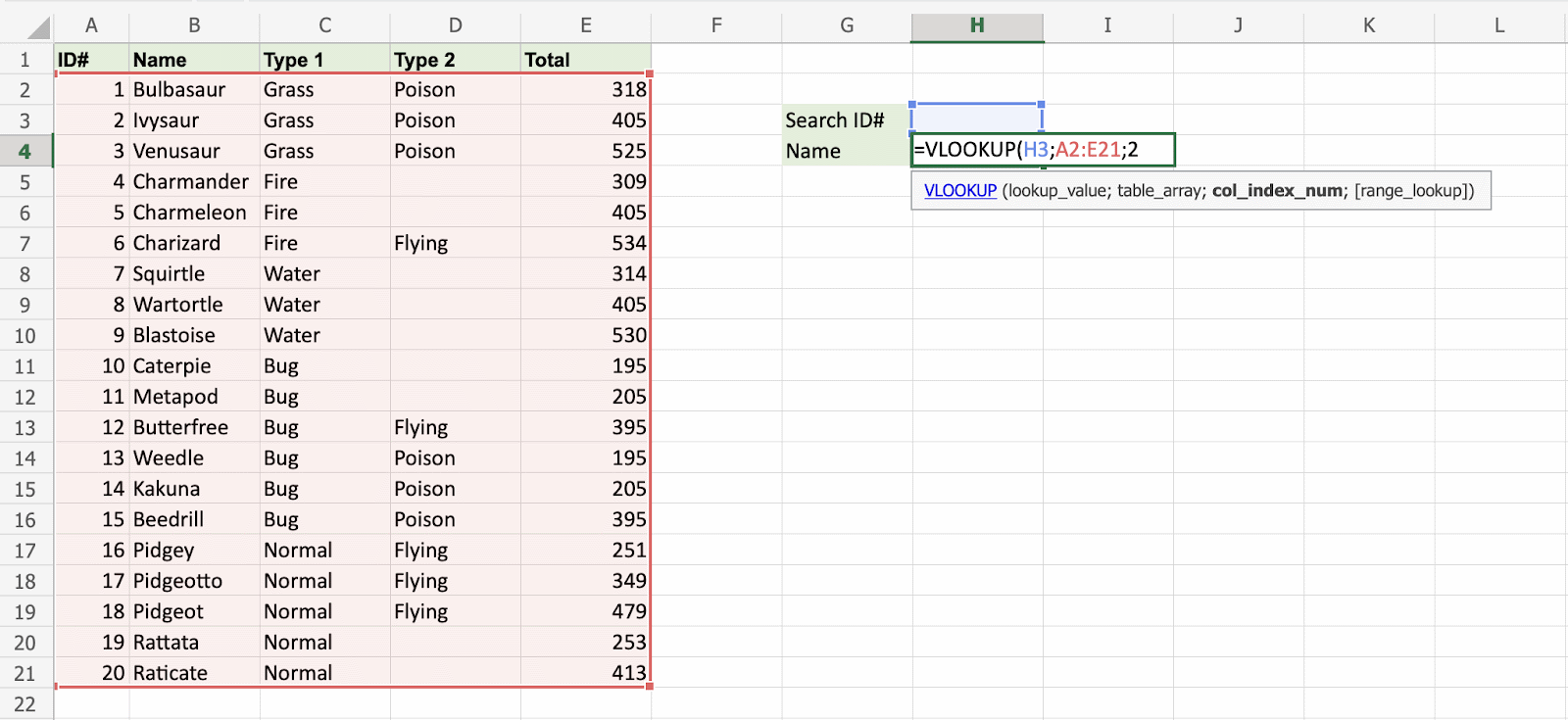The number `2` is entered as col_index_number. This is the second column from the left and is the data that is being looked up.An illustration for selecting col_index_number `2`.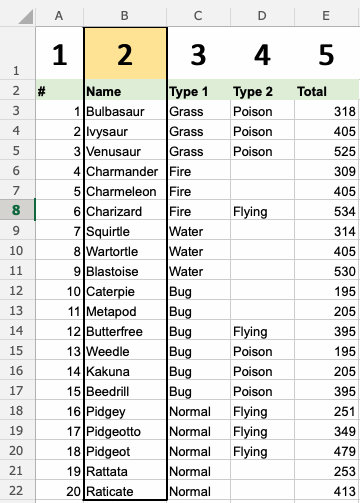Ok, so next - `1` (True) is entered as range_lookup. This is because the most left column has numbers only. If it was text, ``` 0``` (False) would have been used.Good job! The function returns the `#N/A` value. This is because there have not been entered any value to the Search ID# `H3`.

Let us feed a value to it, type `H3(7)`:Have a look at that! The VLOOKUP function has successfully found the Pokemon Squirtle which has the ID# `7`.

One more time, type `(H3)4`: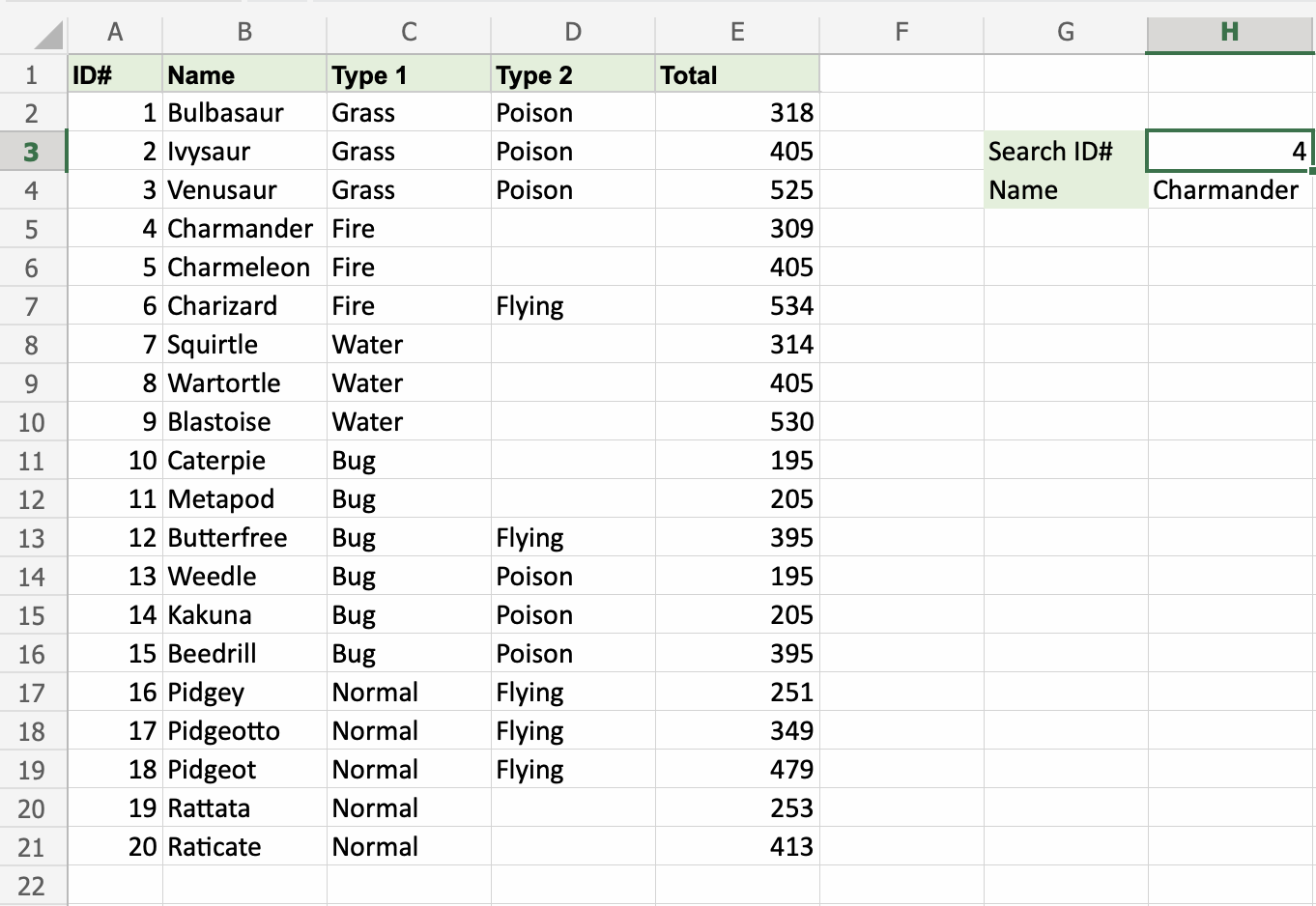It still works! The function returned Charmanders name, which has `4` as its ID#. That's great.

W3Schools is optimized for learning and training. Examples might be simplified to improve reading and learning. Tutorials, references, and examples are constantly reviewed to avoid errors, but we cannot warrant full correctness of all content. While using W3Schools, you agree to have read and accepted our terms of use, cookie and privacy policy.